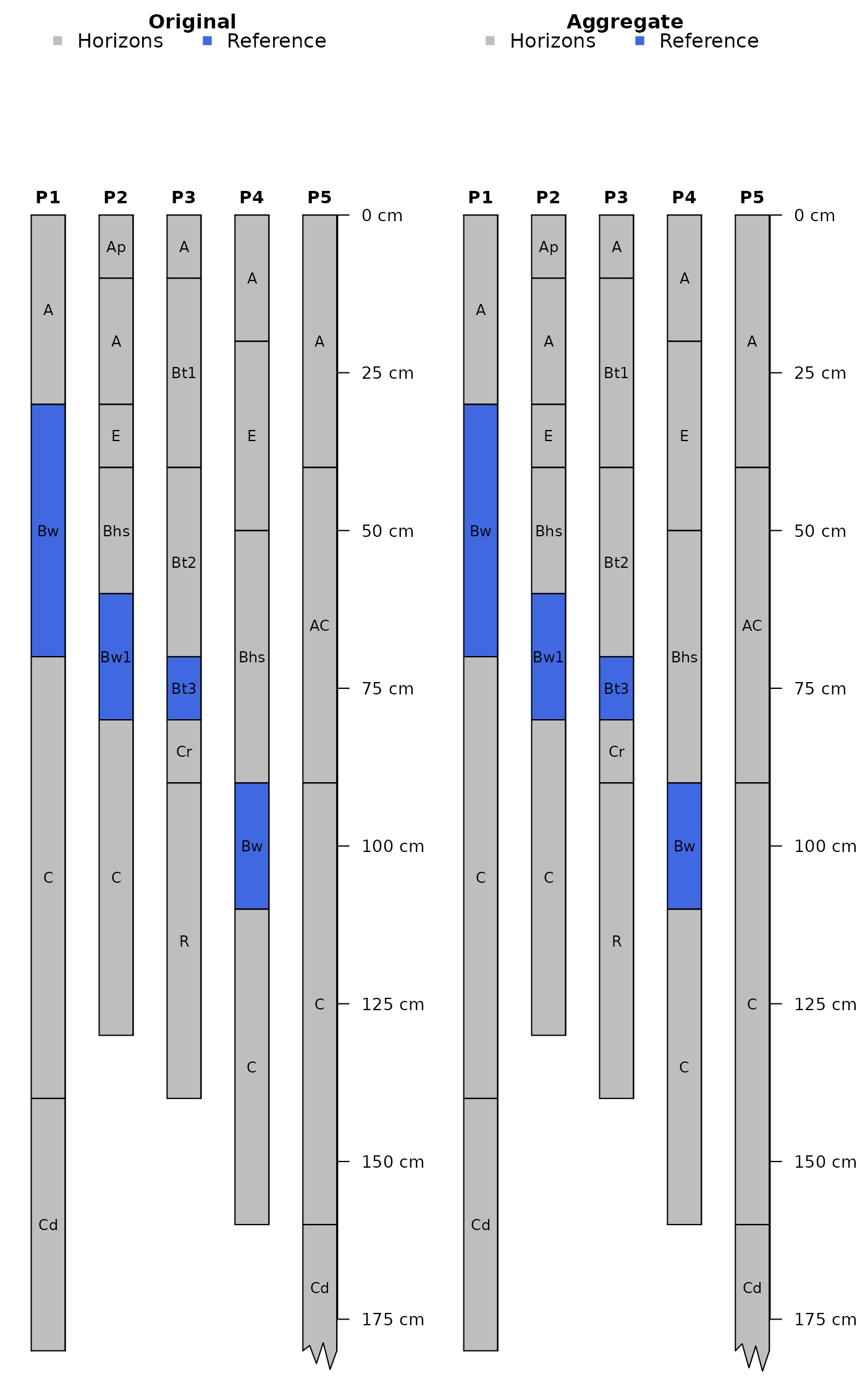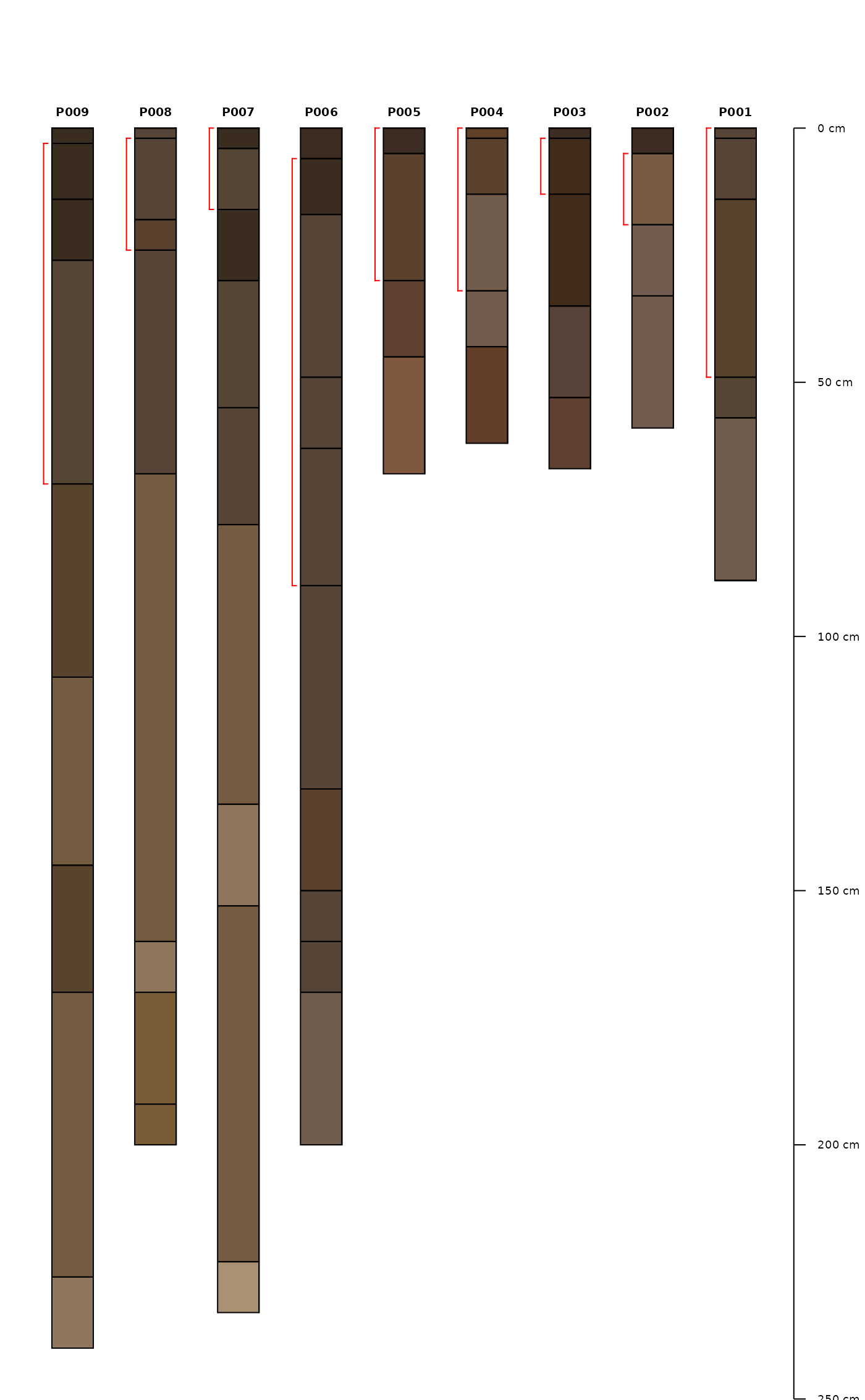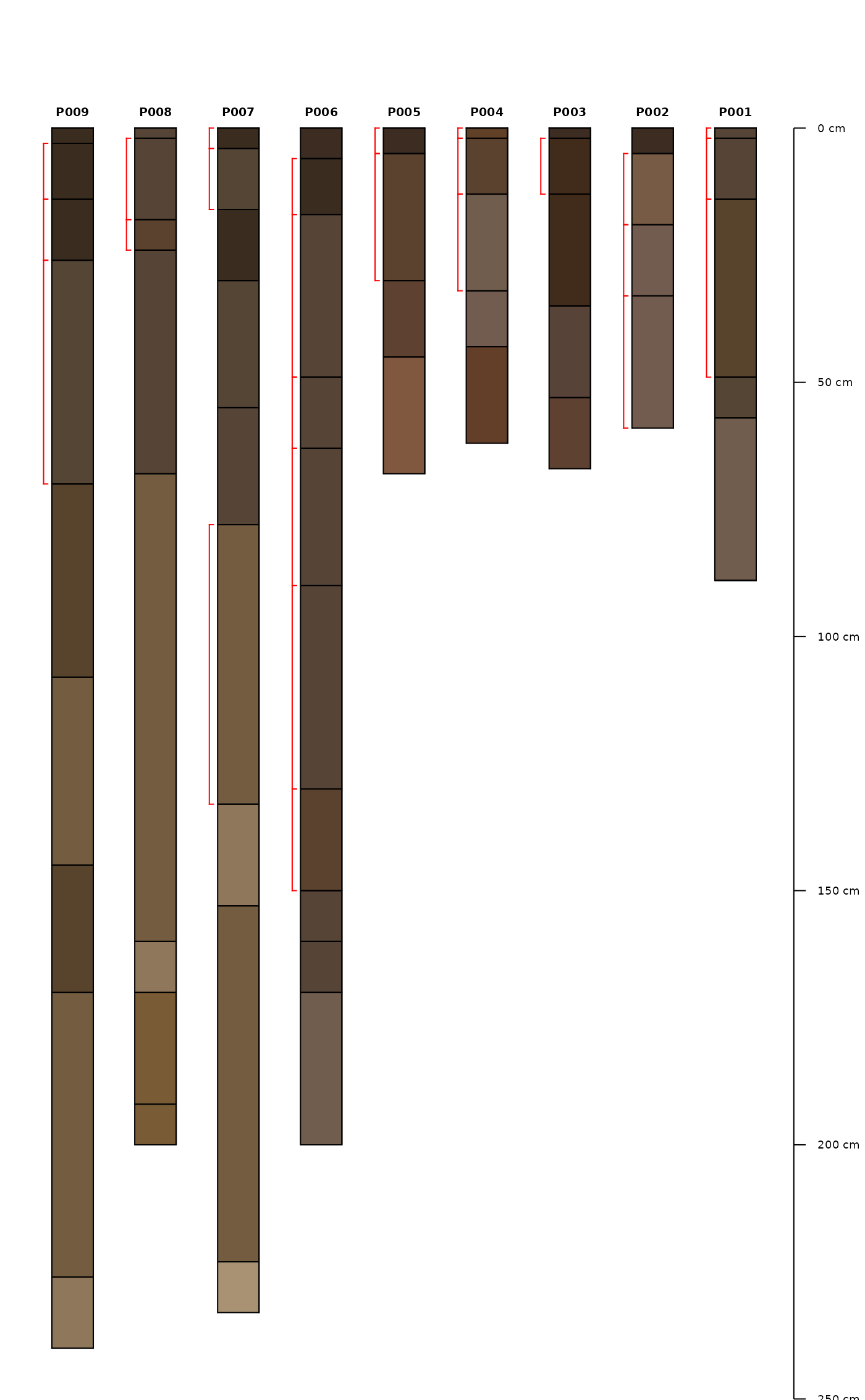Add depth brackets to soil profile sketches.

addBracket(
x,
label.cex = 0.75,
tick.length = 0.05,
arrow.length = 0.05,
offset = -0.3,
missing.bottom.depth = NULL,
...
)

## Arguments

x

data.frame object containing idname(x), top, bottom, and optionally label columns

label.cex

scaling factor for label font

tick.length

length of bracket "tick" mark

arrow.length

offset

left-hand offset from each profile

missing.bottom.depth

distance (in depth units) to extend brackets that are missing a lower depth (defaults to max depth of collection)

...

further arguments passed on to segments or arrows

## Details

x may contain multiple records per profile. Additional examples can be found in this tutorial.

## Note

This is a low-level plotting function: you must first plot a SoilProfileCollection object before using this function.

addDiagnosticBracket, plotSPC

D.E. Beaudette

## Examples


# sample data
data(sp1)

sp1$soil_color <- with(sp1, munsell2rgb(hue, value, chroma)) # promote to SoilProfileCollection depths(sp1) <- id ~ top + bottom # plot profiles par(mar = c(0, 0, 0, 1)) plotSPC(sp1, width = 0.3)# extract min--max depths associated with all A horizons # result is a single-row data.frame / profile combinedBracket <- function(i) { h <- horizons(i) idn <- idname(i) this.id <- h[[idn]] idx <- grep('^A', h$name)

res <- data.frame(
id = this.id,
top = min(h$top[idx]), bottom = max(h$bottom[idx], na.rm=TRUE)
)
names(res) <- idn

return(res)
}

# return matching horizon top / bottom depths for A or C horizons
# result is a 0 or more row data.frame / profile
individualBrackets <- function(i) {
h <- horizons(i)
idn <- idname(i)
this.id <- h[[idn]]

idx <- grep('^A|^C', h$name) res <- data.frame( id = this.id, top = h$top[idx],
bottom = h\$bottom[idx]
)
names(res) <- idn

return(res)
}

# combined brackets
b1 <- profileApply(sp1, combinedBracket, frameify = TRUE)

# individual brackets
b2 <- profileApply(sp1, individualBrackets, frameify = TRUE)

# plot in reverse order
plotSPC(sp1, plot.order = rev(1:length(sp1)), width = 0.25)

# note that plotting order is derived from the call to plotSPC(sp1)# note that plotting order is derived from the call to plotSPC(sp1)